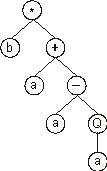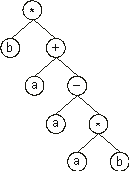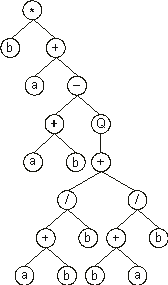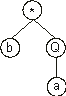Home
News
Author
Q&A
Tutorials
GEP Biblio
Contacts

Visit Gepsoft

Gene Expression Programming: Mathematical Modeling by an Artificial Intelligence

Structural and functional organization of genes

The genes of gene expression programming are composed of a head and a tail. The head contains symbols that represent both functions and terminals, whereas the tail contains only terminals. For each problem, the length of the head h is chosen, whereas the length of the tail t is a function of h and the number of arguments of the function with more arguments n (also called maximum arity) and is evaluated by the equation:

 t = h (n-1) + 1 (2.4)

Consider a gene for which the set of functions F = {Q, *, /, -, +} and the set of terminals T = {a, b}. In this case n = 2; if we chose an h = 15, then t = 15 x (2 - 1) + 1 = 16; thus, the length of the gene g is 15 + 16 = 31. One such gene is shown below (the tail is shown in blue):

 0123456789012345678901234567890 *b+a-aQab+//+b+babbabbbababbaaa (2.5)

It codes for the following ET:Note that the ORF ends at position 7, whereas the gene ends at position 30.

Suppose now a mutation occurred at position 6, changing the “Q” into “*”. Then the following gene is obtained:

 0123456789012345678901234567890 *b+a-a*ab+//+b+babbabbbababbaaa (2.6)

And its expression gives:In this case, the termination point shifts one position to the right (position 8).

Consider another mutation in the chromosome (2.5) above, the substitution of “a” at position 5 by “+”. The following chromosome is obtained:

 0123456789012345678901234567890 *b+a-+Qab+//+b+babbabbbababbaaa (2.7)

Its expression gives:In this case, the termination point shifts twelve positions to the right (position 19).

Obviously the opposite also happens, and the ORF becomes smaller. For instance, suppose a mutation occurred at position 2 in the gene (2.5) above, changing the “+” into “Q”, giving:

 0123456789012345678901234567890 *bQa-aQab+//+b+babbabbbababbaaa (2.8)

Its expression results in the following ET:In this case, the ORF ends at position 3, shortening the original ET in four nodes.

So, despite its fixed length, each gene has the potential to code for ETs of different sizes and shapes, being the simplest composed of only one node (when the first element of a gene is a terminal) and the largest composed of as many nodes as the length of the gene (when all the elements of the head are functions with maximum arity).

It is evident from the examples above, that any modification made in the genome, no matter how profound, always results in a structurally correct expression tree. Obviously, the structural organization of genes must be preserved, always maintaining the boundaries between head and tail and not allowing symbols from the function set on the tail. We will pursue these matters further in the next chapter (section 3.3) where the mechanisms and the effects of different genetic operators are thoroughly analyzed.

Home | Contents | Previous | Next